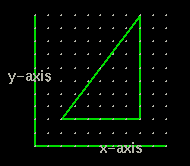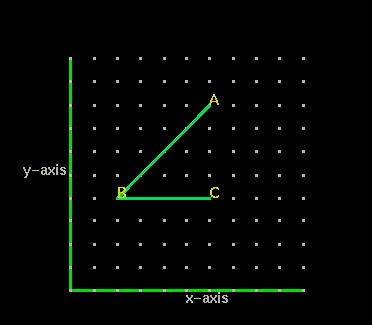## The Geometry of 2 DimensionsWhen we see a graph on a page we really don't think in terms of dimensions. But the graph above has only two axis or two dimensions. It is a plane.

Watch how the x-y graph rotates you can see it is a plane....

-------------->spin on -------->- spin off

Now look at the static graph below with the green axis. Each point is represented by an x and y position. To find the x-position we count to the right. To find the y-position we count up. Angle ABC is represented by three points.Point B has the coordinates (2,4). Starting from the origin (0,0) to reach point B we count 2 spaces to the right, then four spaces up.

What are the coordinates of point A?

.

What are the coordinates of point C?
.

What type of angle is shoTSwn on the graph below?

ADD AN ISOSCLES TRIANGLE WITH QUESIONST